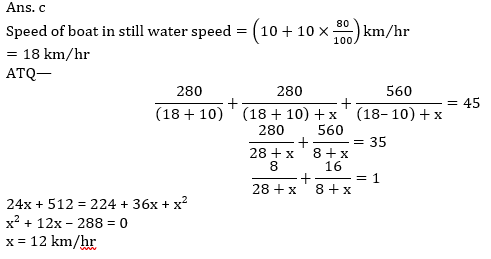# RBI Assistant Pre 2022 Quantitative Aptitude Quiz – 16

## RBI Assistant 2022 Quantitative Aptitude Quiz

Quantitative Aptitude measures a candidate’s numerical ability and problem-solving abilities. It is the most important section of almost all competitive exams. Candidates are often stymied by the complexity of Quantitative Aptitude Questions but if they practice more and more questions, it will become quite simple. So, here we are providing you with the RBI Assistant 2022 Quantitative Aptitude Quiz to enhance your preparation for your upcoming examination. This RBI Assistant 2022 Quantitative Aptitude Quiz is based on the most recent and the latest exam pattern. A detailed explanation for each question will be given in this RBI Assistant 2022 Quantitative Aptitude Quiz. This RBI Assistant 2022 Quantitative Aptitude Quiz is entirely free of charge. This RBI Assistant 2022 Quantitative Aptitude Quiz will assist aspirants in achieving a good score in their upcoming examinations.

Directions (1-5): Given below pie chart shows distribution cars sold by five different companies in 2017. Study the following pie chart and answer the following question given below: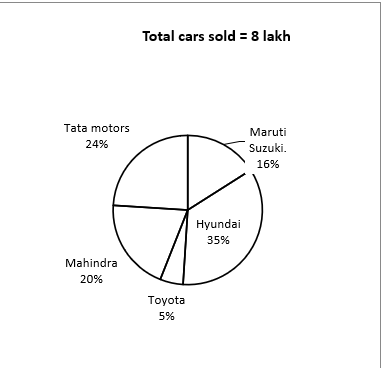1. If Mahindra sold only three types of car ‘XUV 500’, ‘XUV 800’ and ‘XUV 500 PLUS’ in the ratio of 7 : 4 : 5, then find total number of XUV 500 & XUV 500 PLUS sold by Mahindra is what percent of total number of cars sold by Tata Motors?
(a) 67.5%
(b) 62.5%
(c) 57.5%
(d) 52.5%
(e) 47.5%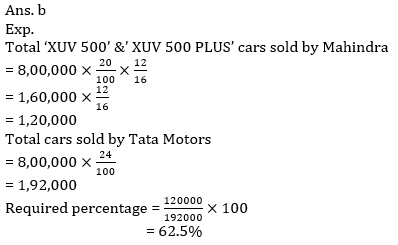2. Out of total cars sold by Hyundai 15% are Verna cars. If 28% of remaining cars are I20, then find the ratio between total Verna and I20 car sold by Hyundai to total car sold by Toyota?
(a) 250 : 679
(b) 697 : 250
(c) 679 : 250
(d) 250 : 667
(e) 250 : 6973. Total cars sold by Mahindra is what percent less than total cars sold by Tata motors?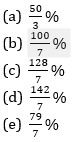4. If total number of cars sold by another company Honda in 2017 is 20% more than total cars sold by Hyundai, then find the average number of cars sold by Honda and Tata Motors together in 2017?
(a) 2,64,800
(b) 2,66,400
(c) 2,65,000
(d) 2,64,400
(e) 2,64,000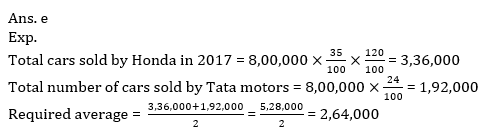5. If 20% of total cars sold by Toyota and 40% of total cars sold by Maruti Suzuki are of white color and remaining are black color cars, then find total black color cars sold by both companies together?
(a) 108000
(b) 108880
(c) 108400
(d) 108800
(e) 106800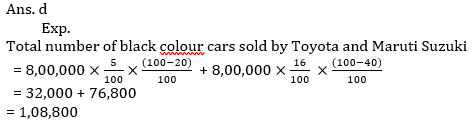6. P and Q started a business together with initial investments of Rs 1500 and Rs 1800 respectively. After some month ‘R’ also joined them with Rs 2400. If total profit after a year was Rs 70,500 and Q’s share in profit is Rs 4500 more than the profit of ‘P’ then find after how many months R joined them.
(a) 7 months
(b) 8 months
(c) 5 months
(d) 6 months
(e) 10 months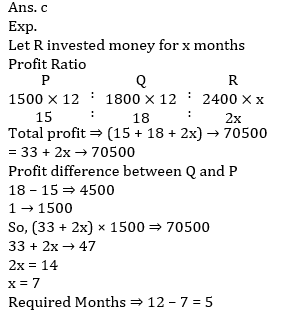7. Ratio between magnitude of volume of a cylinder to the magnitude of curved surface area of the cylinder is 7 : 2. find the total surface area of the cylinder if height of cylinder is double than that of the radius of the cylinder
(a) 968 unit^2
(b) 814 unit^2
(c) 950 unit^2
(d) 616 unit^2
(e) 924 unit^28. The speed of Tejas express and Rajdhani express is 108 km/hr and 144 km/hr respectively. Tejas express crossed stationary Shatabdi express in 52/3 sec. If the ratio of length of Tejas express, Shatabdi express and Rajdhani express is 6 : 7 : 8.Then in what time, Rajdhani express will cross Tejas express if both are running in the same direction?
(a) 48 sec
(b) 56 sec
(c) 42 sec
(d) 40 sec
(e) 36 sec9. There are 3 baskets, each contains three apples and three oranges. Find the probability of selecting two oranges from the same basket?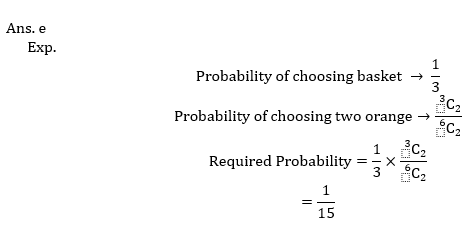10. Speed of current is 10 km/hr and speed of boat in still water is 80% more than the speed of current. A boat travels 50% of total distance of 560 km in downstream, after that the speed of boat is increased by x kmph because he added an engine in the boat. If boat can complete whole journey downstream and upstream in 45 hr, then find the value of x?
(a) 18 km/hr
(b) 10 km/hr
(c) 12 km/hr
(d) 15 km/hr
(e) 14 km/hr